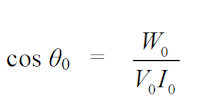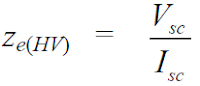# Open Circuit Test and Short Circuit Test on Transformer( SC/OC)

Mainly two tests carried out on the electrical transformer that are open circuit and short circuit test of the transformer also known as SC and OC test.

The purpose of these tests is to determine the parameter of the equivalent circuit, voltage regulation and efficiency of the single / three-phase transformer. this is the main reason for doing SC and OC tests on the transformer. The power required during the test is equal to the power losses occurring in the three-phase transformer.

## Open circuit test of transformer

Circuit diagram for performing OC test also called a no-load test on transformer is shown in the figureConnection diagram open circuit OC test of transformer

In the connection diagram voltmeter, ammeter and wattmeter are connected on the low voltage side of the transformer and HV side is left open-circuited.
Rated frequency voltage is applied to the primary side of the transformer that is low voltage side and this applied voltage is varied with the help of auto transformer. when the voltmeter reading equal to the rated voltage of the LV winding. all meter readings are recorded.

Ammeter records no-load current or exciting current Ie since the no-load current is small, the primary leakage impedance drop is negligible. the applied voltage V1 is equal to the induced EMF V1' .
The input power recorded by the wattmeter consists of core loss and ohmic loss. the no-load current is about 2 to 6% of full load current and ohmic losses in primary of the transformer vary from 0.04% to 0.36% of full load primary ohmic loss.

In fact, ohmic loss during the open-circuit test is negligible as compared to the normal core losses. hence the wattmeter reading can be considered equal to the core losses.

The phasor diagram is shown in the below figurePhasor Diagram of Open Circuit Test

Parameters are obtained from O C test and equivalent circuit diagram

### Calculations of open circuit tests :

Calculation no-load power factor cos θ =Calculation magnetizing current Im

Calculation core loss component of current Ic

Magnetising  reactance Xm (LV)

Resistance representing core loss

## Short circuit test of transformercircuit diagram short circuit test transformer

### Why S.C test is done on the transformer?

The purpose of the test is to find out losses in transformer and equivalent circuit parameters. In the short circuit test of the transformer low voltage side of the transformer is short-circuited and the ammeter, voltmeter, and wattmeter are connected on the HV side. applied voltage to HV side is adjusted by autotransformer.

In the transformer primary side, mmf is almost equal to the secondary mmf , therefore rated current in the primary that is HV winding causes to flow rated current in secondary that is LV winding.
2 to 12% of the rated voltage is sufficient to drive rated current in both primary and secondary winding.

Parameters are obtained from S.C  test & equivalent circuit diagram

### calculations of SC tests :

Equivalent resistance referred to HV side re (HV)Equivalent impedance ref. to HV side ze(HV)Equivalent leakage reactance ref. to HV side xe(HV)

Difference between CT & PT